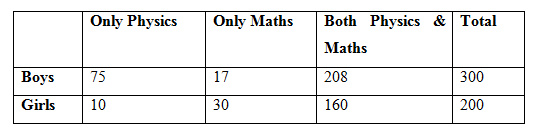# SBI Clerk Prelims 2021 Quantitative Aptitude Questions (Day-23)

Dear Aspirants, Our IBPS Guide team is providing new series of Quantitative Aptitude Questions for SBI Clerk Prelims 2020 so the aspirants can practice it on a daily basis. These questions are framed by our skilled experts after understanding your needs thoroughly. Aspirants can practice these new series questions daily to familiarize with the exact exam pattern and make your preparation effective.

Start Quiz

Caselet

Directions (01-05): Study the following information carefully and answer the questions given below:

In an examination (Consisting two papers Maths and Physics). Total 500 students appeared in the exam. The ratio of boys to girls is 3: 2. The number of boys who passed only in Physics is 25% of the total number of boys and the number of boys who passed only in Physics is 5/2 of the number of girls who passed only in Maths. The number of girls who passed in both the papers is 32 % of the total number of the students and the number of boys who passed in both the papers is 130% of the number of girls who passed in both the papers. None of the candidates failed in both the papers.

1) How many girls are there who passed only in physics paper?

A.11

B.8

C.10

D.12

E.None of these

2) The number of boys who passed only in Maths is what percentage of the total number of students who appeared in the examination?

A.3%

B.3.4%

C.5%

D.2.4%

E.None of these

3) How many students passed in Physics?

A.453

B.385

C.440

D.397

E.None of these

4) What is the ratio of the number of boys who passed in Maths to the number of girls who passed only in Physics?

A.55: 2

B.45: 4

C.45: 2

D.68: 3

E.None of these

5) By what percentage the number of students passed in Physics exam is more or less than the number of students passed in Maths exam?

A.9%

B.25%

C.7%

D.12%

E.20%

Simplification

Directions (06-10): what value should come in place of (?) in the following questions?

6) (9786 + 549) ÷ 65 + 362 + (1/4) of 1680 =?

A.1675

B.1275

C.1875

D.3692

E.1636

7) 1323 ÷ (27 % of 700) = 35 x 7 3/5 of (? ÷190)

A.5

B.7

C.9

D.8

E.10

8) 18 1/2 % of 9800 + 23 1/3 % of 8700 = (5/9) of 810 + ?

A.3093

B.3193

C.2793

D.3293

E.3393

9) (28 x 9 + 54 x 3 + 12 x 11) ÷ (196 – √961 + 17) =?

A.5

B.7

C.4

D.3

E.9

10) (√8 x √8)2 + (9)1/2 = (?)3 + 3

A.4

B.2

C.16

D.5

E.8

Directions (1-5):

Total students in the college = 500

No. of boys = 3/5 x 500 = 300

No. of girls = 500 – 300 = 200

No. of boys passed only in Physics = 25/100 x 300 = 75

No. of girls passed only in Maths = 2/5 x 75 = 30

No. of girls passed in both papers = 32/100 x 500 = 160

No. of boys passed in both papers = 130/100 x 160 = 208

No. of boys passed only in Maths = 300 – (208 + 75) = 17

No. of girls passed only in Physics = 200 – (160 + 30) = 10No. of girls passed only Physics = 200 – (160 + 30) = 10

No. of boys passed only Maths = 300 – (208 + 75) = 17

Total number of students = 500

Percentage % = 17/500 x 100 = 3.4 %

So, total number of students passed in Physics = (75 + 10 + 160 + 208) = 453

No. of boys who passed only in Maths = 17

No. of boys passed in both papers = 130/100 x 160 = 208

Total no. of boys passed in maths = 225

No. of girls passed only in Physics = 10

So, ratio = 225/10 = 45:2

Total no. of students passed in Physics = 10 + 75 + 160 + 208 = 453

Total no. of students passed in Maths = 30 + 17 + 160 +208 = 415

So, percentage % = (453 – 415)/415 x 100 = 9.15 % = 9 approx.

?= (10335/65) + 1296 + 420

?= 159 + 1296 + 420

?= 1875

1323 ÷ (27 x 7) = 35 x (38/5) x (?/190)

1323 ÷ 189 = 35 x (38/5) x (?/190)

? = (7 x 5 x 190)/(35 x 38)

? = 5

(37/200) x 9800 + (70/300) x 8700 = (5/9) x 810 +?

? = 1813 + 2030 – 450

= 3393

? = (252 + 162 + 132) ÷ (196 – 31 + 17)

? = 546/182

? = 3

82 + 3 = (?)3 + 3

(?)= 64

? = 4

 Check Here to View SBI Clerk Prelims 2021 Quantitative Aptitude Questions Day – 22 Day – 21 Day – 20
4 1 vote
Rating